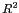DATA MINING
Desktop Survival Guide
by Graham Williams### Discussion

Logistic regression using glm can be applied to tabular data or directly on raw data.

Tabular data partitions the population on each of the variables and then records the count of the two outcomes for each cell (i.e., each possible combination of variables). This table is then passed to glm as the target. An alternative, still using tabular data, is to pass to glm as the target the proportion of entities of interest in each cell, in which case the weight parameter is used to record the population size in each cell.

In data mining though we often have raw data--that is, we have a collection of entities and for each observation the target is recorded. This dataset is provided to glm directly as it is to build a logistic regression model, as we have seen above.

The Design package () provides the lrm function for logistic regression models. The results include a variety of statistics covering, for example, the model likelihood ratio chi-square, the area under the ROC curve (the c index), and the Nagelkerkeindex.

 ```> library(Design) > audit <- read.csv(url("http://rattle.togaware.com/audit.csv")) > mylrm <- lrm(TARGET_Adjusted ~ ., data=audit[,c(2:10,13)]) > mylrm\$stats Obs Max Deriv Model L.R. d.f. P C 1899.0000000 138.2894227 796.4264592 44.0000000 0.0000000 0.8952937 Dxy Gamma Tau-a R2 Brier 0.7905874 0.7914751 0.2847297 0.5156952 0.1081732 ```